DPGraph Documentation Home | Buy | Free Viewer | Legacy Site Licensees  Latest news: 29 Sep 2019 | Update to newest version: 19 Dec 2016  Math Art Gallery | Documentation | Links | Privacy | Contact

After you have downloaded and installed either the full version of DPGraph or the free DPGraph Viewer you can click on the thumbnail pictures to view and manipulate the graphs. If your version of DPGraph or the DPGraph Viewer can't display a graph then you may need to update to the most recent version. To view the commands that created the graphs, click on Edit inside DPGraph or the Viewer. Some versions of Netscape require you to stop and restart your browser after installing.

New! French translations of some of the DPGraph documention, by Laurent Hivon. The files are in pdf format: DPGraph Online Help (in French), by Laurent Hivon and the DPGraph Parametric Graphing Examples (in French), by Laurent Hivon.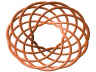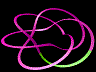Click here for the documentation on parametric curves and surfaces, including examples. Information on creating implicit and vector graphs is below. There are also hundreds of examples in the gallery, and hundreds of examples are included with DPGraph and the DPGraph Viewer.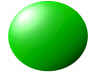DPGraph graphs implicit 3D equations, so the sphere x^2 + y^2 + z^2 = 9 is as easy as GRAPH3D(X^2 + Y^2 + Z^2 = 9) or GRAPH3D( RHO=3 ) in spherical coordinates. No need to solve for z or to use parametric forms.DPGraph can plot multiple equations. To show students three planes intersecting at a point, just GRAPH3D( (X=1, Y=1, Z=1) ).GRAPH3D(SIN(2*X) + SIN(2*Y) = SIN(2*Z)) produces this amazing Schwarz's P surface, contributed by Jerry Glynn.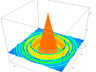Regular z=f(x,y) plots are special cases of implicit plots. Here is GRAPH3D(Z = 6*COS(X^2 + Y^2)*E^(-SQRT(X^2 + Y^2))).3D inequalities are no problem for DPGraph. Graphing |xyz| <= 2 is as easy as GRAPH3D(|X*Y*Z| <= 2). The inequality is true on the "true blue" side, and false on the red side. DPGraph added some blue to the red side, making it more magenta, to show that the inequality is true for the surface itself.Multiple simultaneous 3D inequalities are also easy. Either put them in a list, like the intersecting planes above, or put &'s between them to get a volume of intersection. Here's the inside of a sphere intersected with the outside of a torus: GRAPH3D(X^2 + Y^2 + Z^2 <= 9 & Z^2 + (SQRT(X^2 + Y^2) - 2.5)^2 >= 2).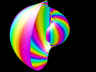You can use the color of the surface to encode an extra dimension of information. This seashell, contributed by Hassan Sedaghat, has been colored by setting GRAPH3D.COLOR := THETA in spherical coordinates. The fractional part of the color expression is mapped to a color wheel that goes from red at 0, through magenta, blue, cyan, green, yellow, and back to red at 1.If you'd like to display the level surfaces (equipotentials, isothermals, etc.) of a function f(x,y,z) at multiples of d, then graph sin(pi*f(x,y,z)/d)=0. For example, here's how to generate the level surfaces of (xy + yz + zx) at multiples of 3.0: GRAPH3D(SIN(PI*((X*Y) + (Y*Z) + (Z*X))/3.0) = 0).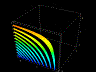One way to make a graph move is to use DPGraph's scrollbar to slice through it in the x, y, or z directions in real time. Here's a movie of the slicer sweeping through the previous level surfaces, showing what the inside of the graph looks like. Inside DPGraph, click on Scrollbar -> X (or Y or Z) slice -> OK.A second way to make a graph move is to use TIME as a variable. Here's a snapshot of the movie GRAPH3D(X^2 + Y^2 + SIN(TIME) = Z^2): a hyperboloid moving from one sheet to two.A third way to make a graph move is to change the variables A, B, C, or D in real time using DPGraph's scrollbar. Here's a snapshot showing the surface of revolution z=x^2+y^2 being generated as A is swept from 0 to 2*pi in GRAPH3D(Z = (X^2+Y^2) * ONE((PI-ANGLE(-X,Y))/A)).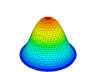A fourth way to make any graph move is to zoom and rotate it using the Home, Page Up, Page Down, and arrow keys. Use the up arrow key to see what's inside this bell curve contributed by Jerry Thornhill.A fifth way to make any graph move is to click on ANIMATE from inside DPGraph and select continuous rotation.A sixth way to make a graph move is to make the color of the surface time-dependent. This sphere has GRAPH3D.COLOR := (SIN(X+TIME)+SIN(Y))/3.In addition to using the slicer, another way to see inside a surface is to make it transparent. This is a movie of a jellyfish head, contributed by Michael Parker.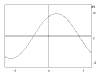You can set DPGraph's parameters to do 2D graphs, too. This graph lets you use DPGraph's scrollbar to change the variables A, B, and C to explore the classic sine curve y=a sin(bx+c) using GRAPH3D((Y = A*SIN(B*X+C), X=0, Y=0)).Notice that the coordinate axes are drawn as the 2D implicit plots x = 0 and y = 0. You can put as many 2D plots as you'd like into the list. For example, here is GRAPH3D((A*X^2+B*Y^2=C^2, X^2=SIN(Y^2), X=0, Y=0)).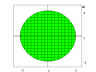2D inequalities are special cases of 3D inequalities, so DPGraph can draw them with ease. For example, to fill the inside of the circle x^2+y^2<6, just GRAPH3D((X^2+Y^2<6 & Z>0, X=0, Y=0 )).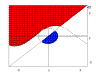Here is a snapshot from a movie of a more complicated set of 2D inequalities: (ydx & y>asin(bx+c) & x^2+y^2>6|sin(t/3)|). While the circle pulses in and out, you can use DPGraph's scrollbar to change A, B, C, and D to vary the parameters for the sine curve and the slope of the line.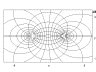As with 3D, DPGraph can display 2D level curves. This is handy for viewing everything from complex variable conformal maps to the characteristic curves of partial differential equations. Here's a snapshot from a movie of equipotential lines "flowing" along streamlines from the source on the right to the sink on the left. You can use DPGraph's scrollbar to adjust A (the distance of the source or the sink from the origin) and C (the strength of the source or the sink).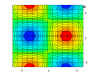Another kind of 2D plot is a contour plot of a 3D graph. Here is a contour plot of z = 3 sin(x) sin(y): GRAPH3D(Z = 3*SIN(X)*SIN(Y)). Red is high in elevation, blue is low.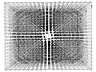DPGraph lets you display 7D graphs (3D arrays of time-dependent 3D vectors). Here is GRAPH3D( VECTOR(-SIN(THETA+TIME)/6, COS(THETA+TIME)/6, 0) ).DPGraph even lets you display 8D graphs -- here is a 3D array of time-dependent 3D vectors, using color as an extra dimension to show a pressure wave propagating through the moving vectors by setting GRAPH3D.VECTORCOLOR := X/6+TIME/6.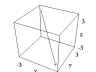If you would only like a few vectors instead of a whole field of them, you can use the NEAR function to select a subset of the vectors. Here is GRAPH3D( VECTOR( NEAR(0,0,0)*A,B,C ) ): a single vector, centered around the origin, that you can move around using the Scrollbar to vary A, B, and C.Ironically, because of its advanced capabilities DPGraph is excellent for demonstrating the beauty of math to beginning students. This stellated octahedron contributed by Владимир Смирнов (Vladimir Smirnov) is an example of what can be done with the absolute value function.Kids don't have to know much math to love using DPGraph. My seven year old son pasted this 3D heart from DPGraph into Greetings Workshop to make his Valentine cards.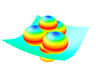Kids love ice cream, too. Here is a bowlful contributed by Сергей Жданов (Sergei Zhdanov).

Dynamic Photorealistic Graphing (DPGraph) -- the world's most powerful 2D through 8D graphing software, optimized for the internet.

Version 2016.12.19. Visit www.dpgraph.com for the full documentation, or click on Open and then Edit to examine the sample files for useful tips; this online help is a summary. Copying DPGraph for unlicensed users is illegal. DPGraph is a trademark of DPGraph. Other company and products names are trademarks or registered trademarks of their respective companies. Copyright (C) 1997-2016 by DPGraph. All rights reserved.

COMMON ERRORS: The seven most common mistakes when creating graphs in the EDIT dialog box are: 1) leaving out asterisks for multiplication; 2) leaving out equals signs or inequality signs; 3) leaving out the parentheses for lists; 4) trying to use equalities in intersections (DPGraph can only intersect inequalities, i.e. regions of space); and 5) putting a space inside the two-character symbols :=, >=, or <=; 6) using the parametric variables u or v in implicit graphs; and 7) using the implicit variables x, y, z, r, theta, rho, or phi in parametric graphs.

 Wrong: Right: `graph3d( 3x = z^2 )` `graph3d( 3*x = z^2 )` `graph3d( x^2+y^2 )` `graph3d( z = x^2+y^2 )` `graph3d( x=1, y=1 )` `graph3d( ( x=1, y=1 ) )` `graph3d( x=1 & y=1 )` `graph3d( x>1 & y>=1 )` `graph3d( z^2> =x^2 )` `graph3d( z^2 >= x^2 )` `graph3d( z=u^2+v^2 )` `graph3d( z=x^2+y^2 )` `graph3d( rectangular(x,y,sin(x+y)) )` `graph3d( rectangular(u,v,sin(u+v)) )`

INEQUALITIES: When graphing an inequality, such as GRAPH3D( X>1 ), remember that "blue is true"; the bluest side of the graph is the region of space for which the inequality is true, the reddest side of the graph is the region of space for which the inequality is false. If the inequality is true for the surface itself, as in GRAPH3D( X>=1 ), then the bluest side is pure blue; if the inequality is false for the surface itself, as in GRAPH3D( X>1 ), then the reddest side is pure red.

DEFAULTS.DPG: With experience you may find the following technique useful when exploring a new surface. OPEN the file DEFAULTS.DPG, go to EDIT, modify the default equation, and then EXECUTE. Since all of the options are already in DEFAULTS.DPG they can be changed with very little typing. If you like, SAVE the modified file under a different name and it will be ready for future viewing and adjustments.

RESOLUTION: The easiest way to improve the appearance of some graphs is to increase the resolution. The default resolution is 21 by 21 by 21 for implicit graphs, and 40 by 40 for parametric surfaces. To change the resolution for implicit graphs, use the EDIT dialog box to insert a line such as the following before the GRAPH3D command:

```graph3d.resolution := 30
```

For parametric surfaces:

```graph3d.stepu := 50
graph3d.stepsv := 50
```

You may find it useful to use the technique described in the previous section on DEFAULTS.DPG.

COPYING A GRAPH TO THE CLIPBOARD: Click on CLIPBOARD to copy a picture of the graph to the clipboard. To copy a picture of the entire window (including the border, etc.) to the clipboard, simultaneously press down the ALT and PRINTSCREEN keys. To copy a picture of the whole screen to the clipboard, press PRINTSCREEN.

COPYING A GRAPH INTO ANOTHER APPLICATION: If the other application supports pasting, first copy the graph to the clipboard. Then click on EDIT in the other application, then click on PASTE.

PRINTING A GRAPH: First copy the the graph to an application, such as Word or Paint, that supports printing. Then click on FILE in the other application, then click on PRINT.

FILE FORMAT: DPGraph files have a file type of .DPG. There are two kinds of DPG files: binary and ASCII. DPGraph can read and write either type of DPG file, but the DPGraph Viewer can only read binary DPG files. Binary DPG files are compressed and contain error detection information for more reliable transmission. ASCII DPG files are readable and editable by Notepad and many other text editors. Each line in the file is a separate command for DPGraph. For example, a file might look like this:

```graph3d.view:=top
graph3d.perspective:=false
graph3d(z=3*sin(x*y))
```

Each command must start on a separate line. The GRAPH3D command must be the last command.

UNINSTALLATION: There are two ways to remove DPGraph from your hard disk drive. Method 1: click on START - SETTINGS - CONTROL PANEL - ADD/REMOVE PROGRAMS, then scroll to DPGraph in the window, select it, and click on ADD/REMOVE. Method 2: click on START - PROGRAMS - DPGRAPH - UNINSTALL.

OPTIONS: Non-numeric default values are given in capital letters.

 `graph3d.resolution` (resolution along longest side of box, default is 21; other sides of box have a resolution proportional to the length of the side) `graph3d.mesh` (TRUE or false) `graph3d.perspective` (TRUE or false) `graph3d.box` (TRUE or false) `graph3d.view` (STANDARD, top, side, front, or textbook) `graph3d.contrast` (default is 1/2, range is 0 to 1) `graph3d.transparency` (default is 0, range is 0 to 1) `graph3d.background` (black, gray, WHITE, brown, red, yellow, green, cyan, blue, or magenta) `graph3d.color` (BYHEIGHT, bysteepness, black, gray, white, brown, red, yellow, green, cyan, blue, magenta, or an expression depending on x, y, z, or time) `graph3d.highlight` (default is 0, range is 0 to 1) `graph3d.shading` (default is 0, range is 0 to 1) `graph3d.vectorcolor` (BYHEIGHT, bysteepness, black, gray, white, brown, red, yellow, green, cyan, blue, magenta, or an expression depending on x, y, z, or time) `graph3d.vectorhighlight` (default is 0, range is 0 to 1) `graph3d.vectorshading` (default is 0, range is 0 to 1) `graph3d.vectoralign` (TAIL, center, or tip) `graph3d.vectorarrowhead` (true or FALSE) `graph3d.minimumx` (default is -3) `graph3d.maximumx` (default is 3) `graph3d.minimumy` (default is -3) `graph3d.maximumy` (default is 3) `graph3d.minimumz` (default is -3) `graph3d.maximumz` (default is 3) `graph3d.minimumu` (default is -3) `graph3d.maximumu` (default is 3) `graph3d.stepsu` (default is 40) `graph3d.minimumv` (default is -3) `graph3d.maximumv` (default is 3) `graph3d.stepsv` (default is 40) `a.minimum` (scrollbar variable minimum value) `a.maximum` (scrollbar variable maximum value) `b.minimum` (scrollbar variable minimum value) `b.maximum` (scrollbar variable maximum value) `c.minimum` (scrollbar variable minimum value) `c.maximum` (scrollbar variable maximum value) `d.minimum` (scrollbar variable minimum value) `d.maximum` (scrollbar variable maximum value)

VALUES FOR OPTIONS:

 `true` (for mesh, perspective, box, and vectorarrowhead) `false` (for mesh, perspective, box, and vectorarrowhead) `standard` (for view, x is the most horizontal axis) `textbook` (for view, y is the most horizontal axis) `top` (for view) `front` (for view) `side` (for view) `byheight` (for color or vectorcolor) `bysteepness` (for color or vectorcolor) `black` (for color, vectorcolor, or background) `gray` (for color, vectorcolor, or background) `white` (for color, vectorcolor, or background) `brown` (for color, vectorcolor, or background) `red` (for color, vectorcolor, or background) `yellow` (for color, vectorcolor, or background) `green` (for color, vectorcolor, or background) `cyan` (for color, vectorcolor, or background) `blue` (for color, vectorcolor, or background) `magenta` (for color, vectorcolor, or background) `tail` (for vectoralign) `center` (for vectoralign) `tip` (for vectoralign)

CONSTANTS:

 `e` (base of natural logarithms) `#e` (base of natural logarithms for Derive users) `pi` (pi)

VARIABLES:

 `x` (x variable for implicit graphing) `y` (y variable for implicit graphing) `z` (z variable for implicit graphing) `r` (polar radius, r=sqrt(x^2+y^2)) `theta` (polar angle, 0<=theta<2*pi, theta=angle(-x,-y)+pi) `rho` (spherical radius, rho=sqrt(x^2+y^2+z^2)) `phi` (spherical angle, 0<=phi<=pi, phi=angle(z,r)) `u` (u variable for parametric graphs) `v` (v variable for parametric graphs) `a` (a variable for scrollbar) `b` (b variable for scrollbar) `c` (c variable for scrollbar) `d` (d variable for scrollbar) `time` (current time for graphs that change with time)

SYMBOLS:

 `=` (equals, for entering equations) `<` (less than, for entering inequalities) `>` (greater than, for entering inequalities) `<=` (less than or equal, for entering inequalities) `>=` (greater than or equal, for entering inequalities) `&` (intersection) `:=` (assignment, for assigning values) `(...)` (lists of arguments or equations) `+` (addition or leading plus sign) `-` (subtraction or leading minus sign) `*` (multiplication) `/` (division) `^` (powers) `|...|` (absolute value, same as abs(...)) `!` (factorial)

ONE ARGUMENT FUNCTIONS:

 `abs` (absolute value, same as |...|) `acos` (arccosine) `acosh` (hyperbolic arccosine) `acot` (arccotangent) `acoth` (hyperbolic arccotangent) `acsc` (arccosecant) `acsch` (hyperbolic arccosecant) `asec` (arcsecant) `asech` (hyperbolic arcsecant) `asin` (arcsine) `asinh` (hyperbolic arcsine) `atan` (arctangent) `atanh` (hyperbolic arctangent) `ceiling` (smallest integer >= argument) `cos` (cosine) `cosh` (hyperbolic cosine) `cot` (cotangent) `coth` (hyperbolic cotangent) `csc` (cosecant) `csch` (hyperbolic cosecant) `floor` (greatest integer <= argument) `gamma` (gamma function) `ln` (log base e) `log` (log base 10) `nonneg` (1 if x>=0, else undefined) `one` (1 if 0<=x<=1, else undefined) `sec` (secant) `sech` (hyperbolic secant) `sign` (-1 if negative, 1 if positive, indefinite if 0 or indefinite) `sin` (sine) `sinh` (hyperbolic sine) `sqr` (square) `sqrt` (square root) `tan` (tangent) `tanh` (hyperbolic tangent)

TWO ARGUMENT FUNCTIONS:

 `angle` (-pi <= angle of (x,y) from positive x axis <= pi) `beta` (beta function) `max` (maximum value) `min` (minimum value)

THREE ARGUMENT FUNCTIONS:

 `cylindrical` (parametric graph in cylindrical coordinates) `near` (1 if (x,y,z) is near current grid, else undefined) `rectangular` (parametric graph in rectangular coordinates) `spherical` (parametric graph in spherical coordinates) `vector` (graphs a vector field)

KNOWN PROBLEMS:

1. Microsoft WordPad under Windows NT 4.0 makes embedded graphs, or graphs copied from the clipboard, too large. It also truncates the graphs on the right hand side.
2. Microsoft Paint in 256 color mode decreases the number of colors in the graphs to 16.